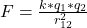## There is a force between two charged objects. To decrease the magnitude of the force, we can There is a force between two charged objects. T

Question

There is a force between two charged objects. To decrease the magnitude of the force, we can There is a force between two charged objects. To decrease the magnitude of the force, we can Move the objects farther from each other or increase the charge on either of them. Move the objects closer to each other or increase the charge on either of them. Move the objects farther from each other or reduce the charge on either of them. Move the objects closer to each other or reduce the charge on either of them.

in progress 0
5 months 2021-08-27T22:51:32+00:00 1 Answers 6 views 0